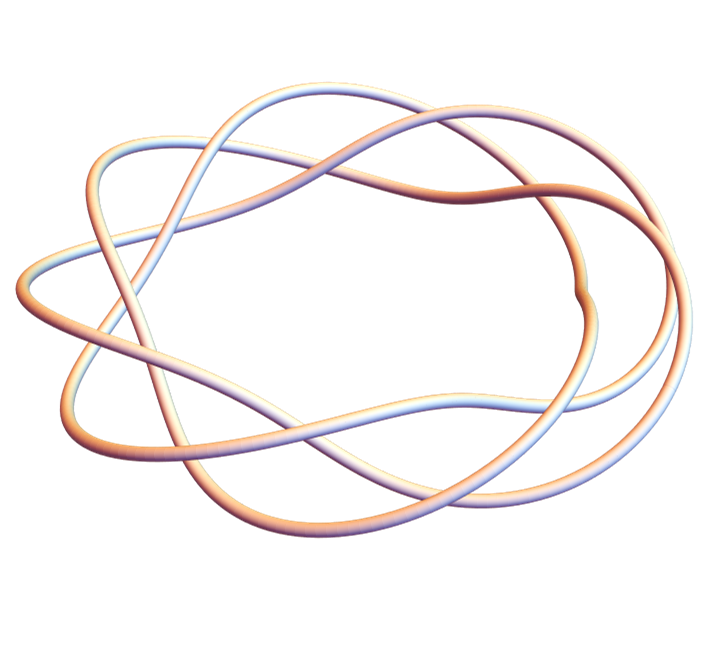# Approximating a golden spiral with circular arcs

The previous post included this image of a logarithm spiral passing through the corners of squares in a sequence of golden rectangles.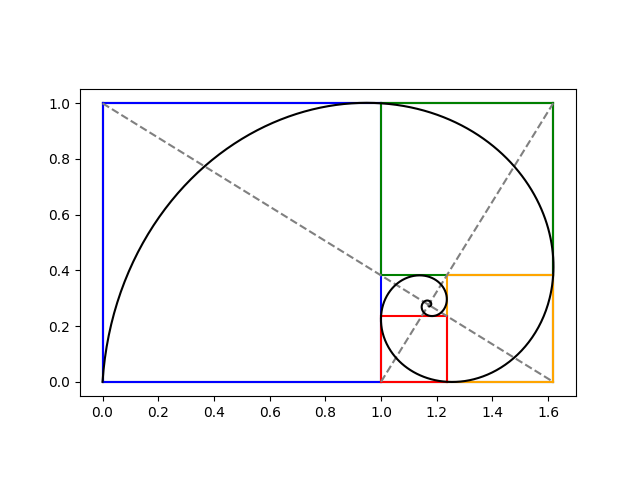The portion of the spiral in each square looks like a quarter of a circle. How well would circular arcs approximate the spiral?

Very well. Here’s a plot.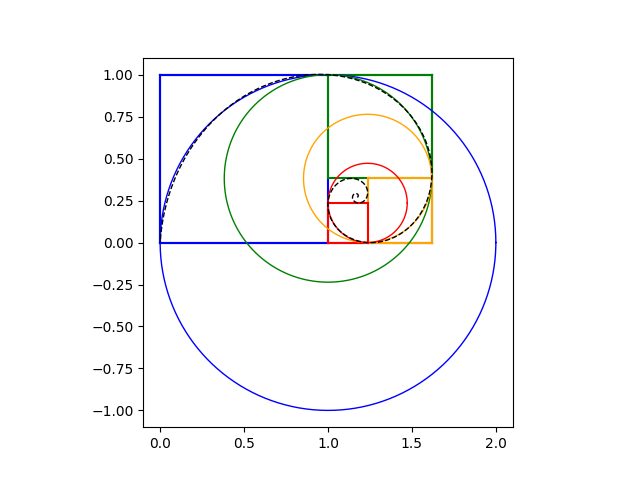The circular arc inside the blue square is plotted in blue, the arc inside the green box in green, etc. The logarithmic spiral is plotted on top with a dashed black line. You have to zoom in closely to see any difference between the logarithmic spiral and its circular approximations.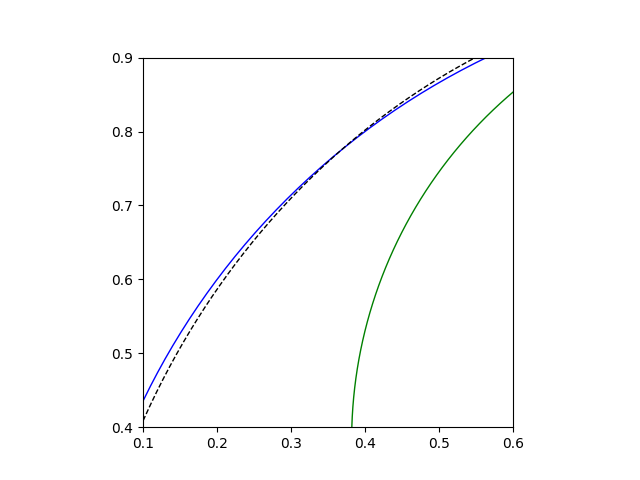For aesthetic applications, circular arcs are good enough and probably easier to work with. For a sort of three-dimensional analog of this approximation, see this post on the geometry of the Sydney Opera House.

Sometimes discontinuities in first or second derivatives are visually noticeable, but here they’re not. The approximation of the logarithmic spiral by a sequence of quarter circles has a jumps in curvature, which is roughly a second derivative. I suppose it’s more noticeable when curvature jumps from positive to zero or from positive to negative. Here it’s jumping from one positive value (the reciprocal of the circle radius) to another positive value (the reciprocal of a smaller radius).

# Area of spherical triangle

A few days ago I wrote about how you could calculate the area of a spherical triangle by measuring the angles at its vertices. As was pointed out in the comments, there’s a much more direct way to find the area.

Folke Eriksson gives the following formula for area in . If a, b, and c are three unit vectors, the spherical triangle formed by their vertices has area E whereLet’s try the formula out with an example and compare the area to that of a flat triangle. We’ll pick three cities as our three points, assuming the earth is a unit sphere.

Let a = Austin, Texas, b = Boston, Massachusetts, and c = Calgary, Alberta. The spherical coordinates of each point will be (1, θ, φ) where θ is longitude and φ is π/2 minus latitude.

    from numpy import *

def latlong_to_cartesian(latlong):
phi   = deg2rad( 90 - latlong )

x = sin(phi) * cos(theta)
y = sin(phi) * sin(thea)
z = cos(phi)
return array([x,y,z])

def spherical_area(a, b, c):
t = abs( inner(a, cross(b, c) ) )
t /= 1 + inner(a,b) + inner(b,c) + inner(a,c)
return 2*arctan(t)

def flat_area(a, b, c):
x = cross(a - b, c - b)
return 0.5*linalg.norm(x)

# Latitude and longitude
austin  = (30.2672,  97.7431)
boston  = (42.3584,  71.0598)
calgary = (51.0501, 114.0853)

a = latlong_to_cartesian(austin)
b = latlong_to_cartesian(boston)
c = latlong_to_cartesian(calgary)

round = spherical_area(a, b, c)
flat  = flat_area(a, b, c)
print(round, flat, round/flat)


This shows that our spherical triangle has area 0.0900 and the corresponding Euclidean triangle slicing through the earth would have area 0.0862, the former being 4.467% bigger.

Let’s repeat our exercise with a smaller triangle. We’ll replace Boston and Calgary with Texas cities Boerne and Carrollton.

    boerne  = (29.7947, 98.7320)
carrollton = (32.9746, 96.8899)


Now we get area 0.000302 in both cases. The triangle is so small relative to the size of the earth that the effect of the earth’s curvature is negligible.

In fact the ratio of the two computed areas is 0.9999, i.e. the spherical triangle has slightly less area. The triangle is so flat that numerical error has a bigger effect than the curvature of the earth.

How could we change our code to get more accurate results for small triangles? That would be an interesting problem. Maybe I’ll look into that in another post.

 “On the measure of solid angles”, F. Eriksson, Math. Mag. 63 (1990) 184–187.

# Triangles on a pseudosphere

The previous post was about triangles on a sphere. This post will be about triangles on a pseudosphere.

A pseudosphere looks something like the bell of a trumpet or a trombone.

Here’s a plot of a pseudosphere.This was created in Mathematica with the code

    ParametricPlot3D[
{
Cos[p] Sech[t],
-Sin[p] Sech[t],
t - Tanh[t]
},
{t,  0, 4},
{p, 0, 2 Pi}
]


The pseudosphere has constant negative curvature just as the sphere has constant positive curvature.

By curvature I mean specifically Gaussian curvature, the product of the sectional curvature in orthogonal directions at each point. A sphere curves the same way in all directions. A pseudosphere curves opposite ways in different directions. Coordinate lines through a point are convex in one direction and concave in another. Their sectional curvatures have opposite signs and so their product is negative.

A spherical triangle is formed by great circles connecting the vertices of the triangles. Great circles are the geodesics on a sphere.

A pseudospherical triangle is a triangle formed by geodesics on the pseudosphere. These are the “straight” lines in the hyperbolic geometry of the surface.

On a sphere, the interior angles of a triangle add up to more than π, and in fact the amount by which the sum of the angles exceeds π is proportional to the area of the triangle.

On a pseudosphere, the interior angles of a triangle add up to less than π. And just as in the case of the sphere, the difference between the sum of the angles and π, now negative, is proportional to the area. This result was discovered by Johann Heinrich Lambert (1728–1777).

On a sphere or a pseudosphere, the interior angles of a small triangle add up to nearly π. As triangles get larger, the sum increases on a sphere but decreases on a pseudosphere.

The general principle behind both of these cases is the local Gauss-Bonnet theorem. For any surface, let T be triangle whose sides are geodesics. Then the integral of the Gaussian curvature over T is equal to the sum of the interior angles of T minus π.

When a surface has constant curvature, the integral of curvature over a region is proportional to the area of the region. So on a sphere or pseudosphere, the area of a geodesic triangle is proportional to the sum of the interior angles minus π.

The local Gauss-Bonnet theorem holds when the sides of a triangle are not geodesics, but in that case the theorem has an extra term, the integral of the geodesic curvature along the sides. Since the geodesic curvature of a geodesic is zero, this term drops out for geodesic triangles.

# Visual geometry

If you’re puzzled by the title of this post, allow me to explain.

A natural reaction would be “Isn’t geometry intrinsically visual?” Indeed, geometry is motivated by things we can visualize. But modern developments of geometry have become heavy with formal machinery, so much so that one could reasonably ask “What happened to the geometry?”

Tristan Needham has a new book entitled Visual Differential Geometry and Forms that aims to put the geometry back into a first course on differential geometry. I expect it’s a good read based on having read his previous book Visual Complex Analysis.

I just got a review copy in the mail, and flipping through the book I can see that it lives up to its title. It has lots of illustrations, just as you’d expect from a book on differential geometry if you hadn’t taken courses in the subject.# Calculating dogleg severity

Dogleg severity (DLS) is essentially what oilfield engineers call curvature.

Oil wells are not simply vertical holes in the ground. The boreholes curve around underground, even if the intent is to drill a perfectly straight hole. With horizontal drilling the curvature can be substantial.

Dogleg severity is calculated by measuring inclination and azimuth every 100 feet along a well. Inclination is the angle the hole makes with the vertical axis, like the angle φ in spherical coordinates, 90° minus the latitude. Azimuth is the angle of the projection of the well to a horizontal plane, like the θ or longitude angle in spherical coordinates. So if a well is nearly vertical, the inclination angles will be small. If a hole were shaped like a corkscrew, the inclination would be constant while the azimuth makes multiple rotations.

When I said 100 feet along the well, that is 100 feet of arc length. If a hole were perfectly vertical, this would be a change of 100 vertical feet, but generally it is less. If a segment were perfectly horizontal, it would be a change of 100 horizontal feet with no change in vertical depth.

Dogleg severity models a section of an oil well as a piece of a big circle. We assume our two inclination and azimuth measurements taken at two points along this circle, separated by an arc of 100 feet. If this arc makes an angle ψ, then the length of the arc is

ρ ψ = 100 ft

where ρ is the radius of the circle. Engineers call the angle ψ the dog leg angle, the angle of the sector between two measurements. Mathematicians call 1/ρ the curvature, so curvature is proportional to DLS.

To calculate curvature or DLS you have to calculate ψ from the two inclination and azimuth readings, (θ1, φ1) and (θ2, φ2). This is exactly the same as calculating distance from longitude and latitude. Instead of longitude and latitude on the earth, imagine a large sphere tangent to the wellbore at the two points where the measurements were taken. To put it another way, we imagine this section of the well as being a piece of a great circle on this sphere.

The only difference is that instead of the radius ρ being fixed and solving for distance, as in the longitude and latitude problem, our distance is fixed at 100 ft and we want to calculate ρ, or equivalently calculate ψ. From these notes we have

ψ = cos-1(cos φ1 cos φ2 + sin φ1 sin φ2 cos(θ12)).

So, for example, suppose we had inclination 4° and azimuth 30° at one point, and inclination 7° and azimuth 40° at the next measurement, 100 ft of arc length away. Then

ψ = cos-1(cos 4° cos 7° + sin 4° cos 7° (cos40° – 30°)) = 3.138°

This says ρ = 1826 feet, i.e. our section of the well is curved like a circle of radius 1826 feet.

We could compute this in Python as follows.

    from numpy import sin, cos, arccos, deg2rad, rad2deg

def dls(inc1, az1, inc2, az2):
cos(ph1)*cos(ph2) + sin(ph1)*sin(ph2)*cos(th1-th2)) )


Here we assume input and output are in degrees, but internally we do calculations in radians.

If we call dls(4, 30, 7, 40) we get back 3.138°.

# Kissing circle

Curvature is a measure of how tightly a curve bends. A circle of radius r has curvature 1/r. So a small circle has high curvature and a big circle has small curvature.

In general the curvature of a curve at a point is defined to be the curvature of the circle that best fits at that point. This circle is called the osculating circle which means the circle that “kisses” the curve at that point.

osculate (v.) “to kiss (one another),” 1650s, from Latin osculatus, past participle of osculari “to kiss,” from osculum “a kiss; pretty mouth, sweet mouth,” literally “little mouth,” diminutive of os “mouth”

The center of the osculating circle is called the center of curvature and the radius of the circle is called the radius of curvature.

I’ll give two examples. The first is a set of ellipses that all have the unit circle as their osculating circle on the left end. So they all have curvature 1.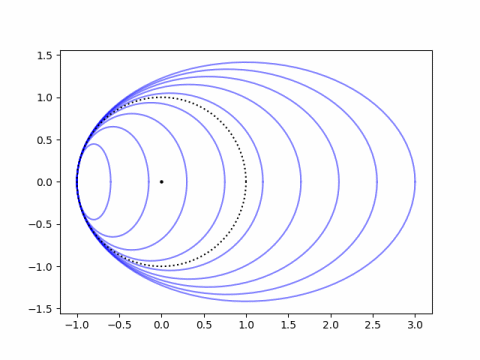An ellipse with equationhas varying curvature at each point. At x = –a the curvature simplifies to a/b². So to make the graph above, I used a range of values for a and set each corresponding value of b to √a.

Next, instead of fixing the osculating circle I’ll fix the curve. I’ll use the equation to fit an egg that I’ve used before and plot the osculating circles at each end. The plot below uses a = 3, b = 2, and k = 0.1.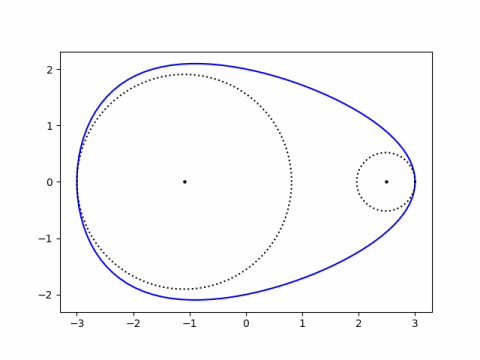The curvature at the fat end is a(1-ka)/b² and the curvature at the pointy end is a(1+ka)/b². These are derived here.

Setting k = 0 gives the curvature of an ellipse at each end used above.

# Surface of revolution with minimum area

Suppose you’re given two points (x1, y1) and (x2, y2) with y1 and y2 positive. Find the smooth positive curve f(x) that passes through the two points such that the area of the surface formed by rotating the graph of f around the x-axis is minimized.

You can state this as a problem in calculus of variations, which leads to a differential equation, which leads to the solution

f(x) = c cosh((x + d)/c).

In other words, the surface area is minimized when the graph of f is a piece of a catenary .

This is interesting because the answer is not something you’re likely to guess, unlike say the isoperimetric problem, where the it’s easy to guess (but hard to prove) that the solution is a circle.

There’s also some interesting fine print to the solution. It’s not quite right to say that the solution is a catenary. To be more precise we should say that if there is a unique catenary that passes through both specified points, then it is the smooth curve with minimal area when rotated about the x-axis. But there are a couple things that could go wrong.

It’s possible that two catenaries pass through the given points, and in that case one of the catenaries leads to minimal surface area. But it’s also possible that there is no catenary passing through the given points.

My first thought would be that you could always find values of c and d so that the function f passes through the points (x1, y1) and (x2, y2), but that’s not true. Often you can, but if the difference in the y‘s is very high relative to the difference in the x‘s it might not be possible.

Suppose the graph of f passes through (0, 1) and (1, y2).

Since the graph passes through the first point, we have

c cosh(d/c) = 1.

Since cosh(x) ≥ 1, we must also have c ≤ 1. And since our curve is positive, we must have c > 0. We can maximize

c cosh((1 + d)/c)

for 0 < c ≤ 1 subject to the constraint

c cosh(d/c) = 1

to find the maximum possible value of y2. If we ask Mathematica

    NMaximize[
{   c Cosh[(1 + d)/c],
{0 < c <= 1},
{c Cosh[d/c] == 1}
},
{c, d}
]


we get

    {6.45659*10^8, {c -> 0.0352609, d -> -0.142316}}

meaning the largest possible value of y2 is 6.45659 × 108, and it occurs when c = 0.0352609, d = -0.142316.

Update: See the comment by Bill Smathers below arguing that the maximum should be unbounded. If the argument is correct, this would imply the code above ran into a numerical limitation.

## Related posts

 See Calculus of Variations by I. M. Gelfand and S. V. Fomin.

# Self-curvature

If you look at a sine wave, its curvature is sorta like its values. When sine is zero, the curve is essentially a straight line, and the curvature is zero. When sine is at its maximum, the function bends the most and so the curvature is at a maximum.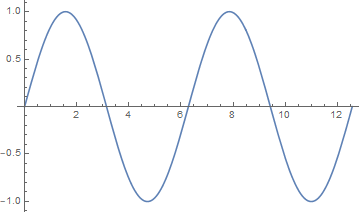This makes sense analytically. The second derivative is something like curvature, and the second derivative of sin(x) is -sin(x). The negative sign suggests that if we look at signed curvature rather than absolute curvature, then the values of a sine curve are roughly proportional to the negative of the curvature at each point.

Here’s a plot showing sin(x) and its negative curvature.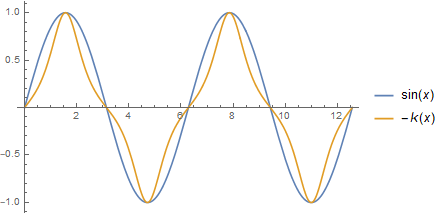Steven Wilkinson wrote an article  addressing the question of whether there is a curve so that the curvature at each point is proportional to the value of the curve. The curvature of a graph of y = y(x) isand so a curve that is proportional to its curvature is a solution to the nonlinear differential equationGeneral theory says that there should at least be a solution in a (possibly small) neighborhood of 0, but it’s not obvious a priori that interesting solutions exist. It turns out that if we pick the proportionality constant a = -1 and the initial conditions y(0) = 0 and y ‘(0) = √3 then we get a periodic solution that looks something like a sine.

The following Mathematica code will plot the function for us.

    s = NDSolve[
{y''[x] = -y[x] (1 + y'[x]^2)^(-3/2),
y == 0, y' == Sqrt},
y, {x, 0, 10}]

Plot[Evaluate[{y[x] /. s}], {x, 0, 10}]


The plot looks a lot like a sine wave. In fact, I imagine that when asked to draw a sine wave, most people would draw something that looks more like this than like an actual sine wave.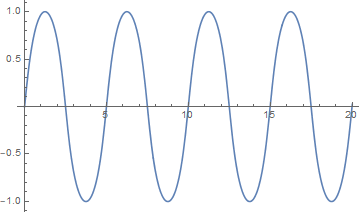## Related posts

 Steven Wilkison. Self-Curvature Curves. Mathematics Magazine, Vol. 82, No. 5 (December 2009), pp. 354-359

# Curvature of an ellipsoid

For an ellipsoid with equationthe Gaussian curvature at each point is given byNow suppose abc > 0. Otherwise relabel the coordinate axes so that this is the case. Then the largest curvature occurs at (±a, 0, 0), and the smallest curvature occurs at (0, 0, ±c).

You could prove this using algebra by manipulating inequalities, or using calculus with Lagrange multipliers.

To see intuitively why this might be true, it helps to exaggerate the shape. First imagine that a is much larger than b or c. Then you have a cigar shape, the greatest curvature as at the two ends. And If you imagine c being much smaller than a and b, you have sort of a pancake shape which is flat on top and bottom.

The maximum curvature is (a/bc)² and the minimum curvature is (c/ab)².

# Total curvature of a knot

Tie a knot in a rope and join the ends together. At each point in the rope, compute the curvature, i.e. how much the rope bends, and integrate this over the length of the rope. The Fary-Milnor theorem says the result must be greater than 4π. This post will illustrate this theorem by computing numerically integrating the curvature of a knot.

If p and q are relatively prime integers, then the following equations parameterize a knot.

x(t) = cos(pt) (cos(qt) + 2)
y(t) = sin(pt) (cos(qt) + 2)
z(t) = -sin(qt)

This is in fact a torus knot because the curve stays on the surface of a torus (doughnut) . We can get a trefoil knot, for example, by setting p = 2 and q = 3.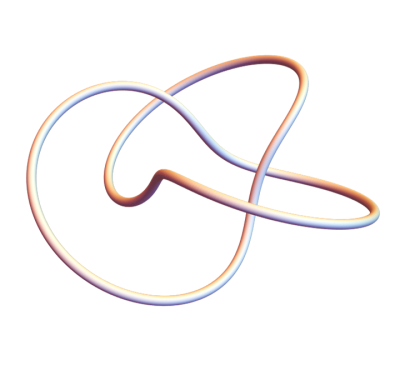We’ll use Mathematica to compute the total curvature of this knot and other examples. First the parameterization:

    x[t_, p_, q_] := Cos[p t] (Cos[q t] + 2)
y[t_, p_, q_] := Sin[p t] (Cos[q t] + 2)
z[t_, p_, q_] := -Sin[q t]


We can plot torus knots as follows.

    k[t_, p_, q_] := {
x[t, p, q],
y[t, p, q],
z[t, p, q]
}
Graphics3D[Tube[Table[k[i, p, q], {i, 0, 2 Pi, 0.01}],
0.08], Boxed -> False]


Here’s a more complicated knot with p = 3 and q = 7.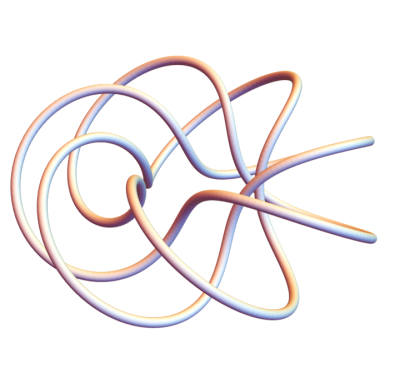Before we can integrate the curvature with respect to arc length, we need an expression for an element of arc length as a function of the parameter t.

    ds[t_, p_, q_] :=  Sqrt[
D[x[t, p, q], t]^2 +
D[y[t, p, q], t]^2 +
D[z[t, p, q], t]^2
]


Now we can compute the total curvature.

    total[p_, q_] := NIntegrate[
ArcCurvature[k[t, p, q], t] ds[t, p, q],
{t, 0, 2 Pi}
]


We can use this to find that the total curvature of the (2,3) torus knot, the trefoil, is 17.8224, whereas 4π is 12.5664. So the Fary-Milnor theorem holds.

Let’s do one more example, this time a (1,4) knot.You can see that this is not actually knot. This doesn’t contradict what we said above because 1 divides 4. When p or q equal 1, we get an unknot.

When we compute its total curvature we get 24.2737, more than 4π. The Fary-Milnor theorem doesn’t say that total curvature in excess of 4π is a sufficient condition for a loop to be knotted; it says it’s necessary. Total curvature less than 4π proves that something isn’t a knot, but curvature greater than 4π doesn’t prove anything.

## More on curvature and knots

 If you change out the 2s in the parameterization with a larger number, it’s easier to see from the graphs that the curves are on a torus. For example, if we plot the (3,7) knot again, replacing 2’s in the definition of x(t) and y(t) with 5’s, we can kinda see a torus inside the knot.